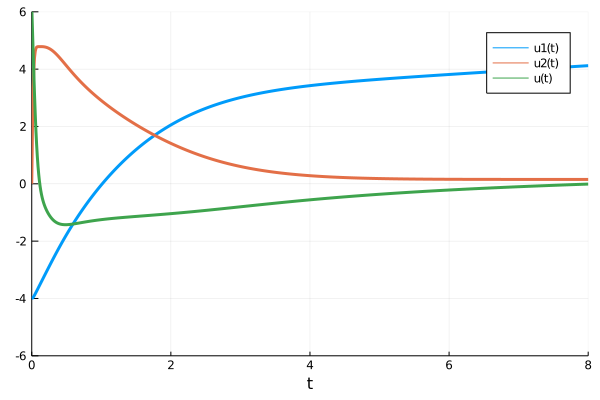# Solving Optimal Control Problems with Universal Differential Equations

Here we will solve a classic optimal control problem with a universal differential equation. Let

$$$x^{′′} = u^3(t)$$$

where we want to optimize our controller u(t) such that the following is minimized:

$$$L(\theta) = \sum_i \Vert 4 - x(t_i) \Vert + 2 \Vert x^\prime(t_i) \Vert + \Vert u(t_i) \Vert$$$

where $i$ is measured on (0,8) at 0.01 intervals. To do this, we rewrite the ODE in first order form:

\begin{aligned} x^\prime &= v \\ v^′ &= u^3(t) \\ \end{aligned}

and thus

$$$L(\theta) = \sum_i \Vert 4 - x(t_i) \Vert + 2 \Vert v(t_i) \Vert + \Vert u(t_i) \Vert$$$

is our loss function on the first order system. We thus choose a neural network form for $u$ and optimize the equation with respect to this loss. Note that we will first reduce control cost (the last term) by 10x in order to bump the network out of a local minimum. This looks like:

using Flux, DifferentialEquations, Optimization, OptimizationOptimJL, OptimizationFlux,
SciMLSensitivity, Zygote, Plots, Statistics, Random

rng = Random.default_rng()
tspan = (0.0f0,8.0f0)
ann = Flux.Chain(Flux.Dense(1,32,tanh), Flux.Dense(32,32,tanh), Flux.Dense(32,1))
θ, re = Flux.destructure(ann)
function dxdt_(dx,x,p,t)
x1, x2 = x
dx = x
dx = re(p)([t])^3
end
x0 = [-4f0,0f0]
ts = Float32.(collect(0.0:0.01:tspan))
prob = ODEProblem(dxdt_,x0,tspan,θ)
solve(prob,Vern9(),abstol=1e-10,reltol=1e-10)

end
mean(abs2,4.0 .- x[1,:]) + 2mean(abs2,x[2,:]) + mean(abs2,[first(re(θ)([t])) for t in ts])/10
end

callback = function (θ,l; doplot=false)
println(l)

if doplot
p = plot(solve(remake(prob,p=θ),Tsit5(),saveat=0.01),ylim=(-6,6),lw=3)
plot!(p,ts,[first(re(θ)([t])) for t in ts],label="u(t)",lw=3)
display(p)
end

return false
end

# Display the ODE with the current parameter values.

callback(θ,l)

# Setup and run the optimization

optprob = Optimization.OptimizationProblem(optf, θ)
res1 = Optimization.solve(optprob, ADAM(0.005), callback = callback,maxiters=100)

optprob2 = Optimization.OptimizationProblem(optf, res1.u)
res2 = Optimization.solve(optprob2,
BFGS(), maxiters=100,
allow_f_increases = false)

Now that the system is in a better behaved part of parameter space, we return to the original loss function to finish the optimization:

function loss_adjoint(θ)
mean(abs2,4.0 .- x[1,:]) + 2mean(abs2,x[2,:]) + mean(abs2,[first(re(θ)([t])) for t in ts])
end

optprob3 = Optimization.OptimizationProblem(optf3, res2.u)
res3 = Optimization.solve(optprob3,
BFGS(),maxiters=100,
allow_f_increases = false)

Now let's see what we received:

l = loss_adjoint(res3.u)
callback(res3.u,l)
p = plot(solve(remake(prob,p=res3.u),Tsit5(),saveat=0.01),ylim=(-6,6),lw=3)
plot!(p,ts,[first(re(res3.u)([t])) for t in ts],label="u(t)",lw=3)